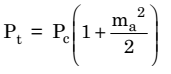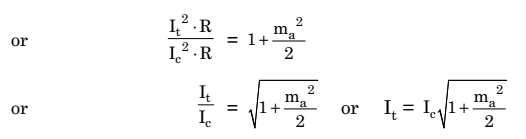# Current Calculation For Single-Tone AM Signal

## Current Calculation For Single-Tone AM Signal

In AM, it is generally more convenient to measure the AM transmitter current than the power.

In this case, the modulation index may be calculated from the values of unmodulated and modulated currents in the AM transmitter.

### Mathematical Expression

Let Ibe the r.m.s. value of the carrier or unmodulated current and It be the r.m.s. value of the total or modulated current of an AM transmitter.

Let R be the antenna resistance through which these currents flow.

Now, we know that for a single-tone modulation the power relation is expressed as :……………….. (1)

where Pt = total or modulated power

Pc = carrier or unmodulated power

ma = modulation index

Now, equation (1) can be written as :